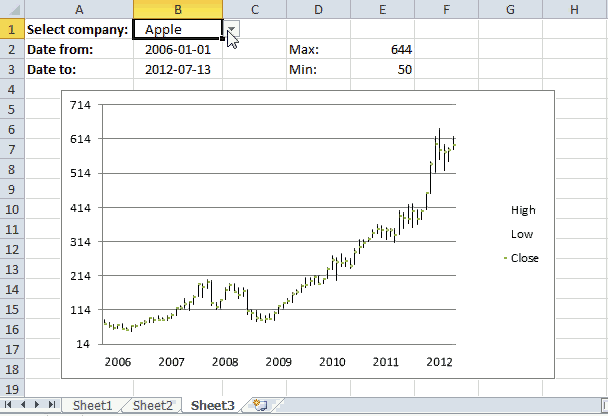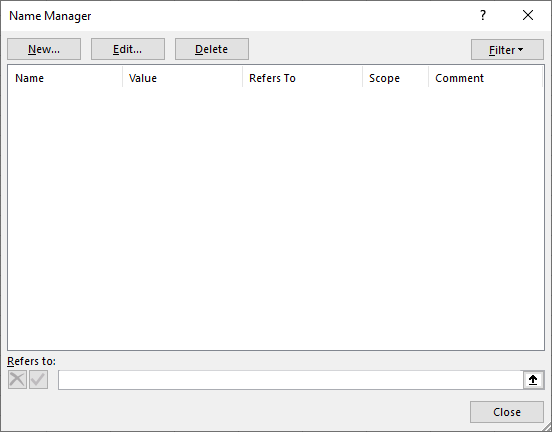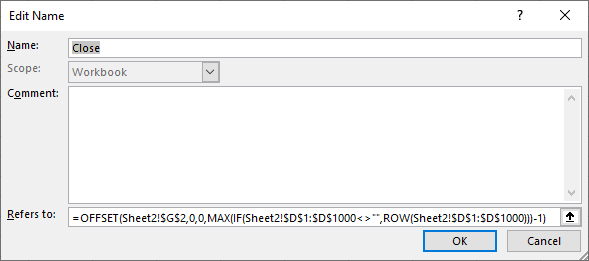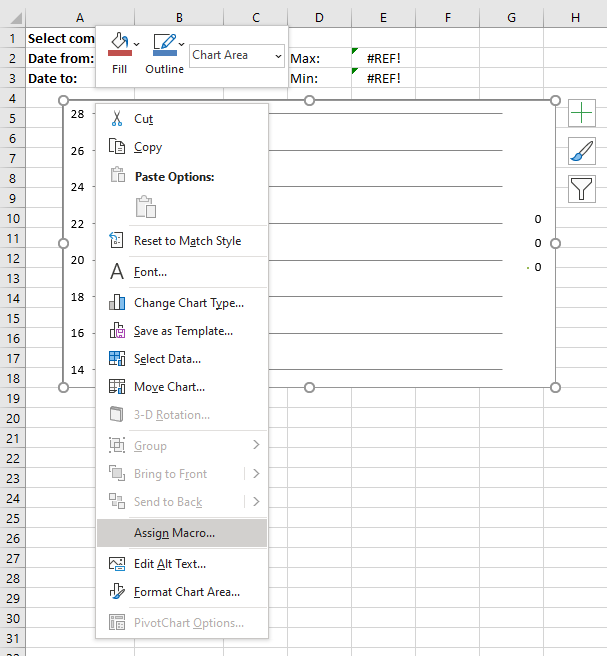Author: Oscar Cronquist Article last updated on September 09, 2019This article demonstrates a macro that changes y-axis range programmatically, this can be useful if you are working with stock charts and you are not happy with the axis range Excel has chosen for you.

The macro automates this for you, the formulas in cell E2 and E3 extracts the largest and smallest stock price respectively. The macro simply uses those values to change the y-axis range for you, you don't need to change these values manually each time you change the data source (stock ticker).

### What you will learn in this article

• Extract the largest and smallest number from a cell range.
• Round a number up and down to the nearest integer.
• Create named range that expands based on size of the data source.
• Change chart axis range programmatically.
• How to use the minimumScale and maximumScale property
• Assign a macro to a chart allowing the user to click the chart in order to run the macro.

Formula in cell E2:

=ROUNDUP(MAX(High),0)

High is a named range that expands based on the size of the data source, it references the column that contains the highest stock price for a given period.

The MAX function returns the maximum number and the ROUNDUP function rounds the number up to the nearest integer.

Formula in cell E3:

=ROUNDDOWN(MIN(Low),0)

Low is a named range that expands based on the size of the data source, it references the column that contains the lowest stock price for a given period.

The MIN function returns the minimum number and the ROUNDDOWN function rounds that number down to the nearest integer.

### How to use named ranges?

1. Click on tab "Formulas" on the ribbon.
2. Click on "Name Manager".3. Click on "New" button.4. Type the name and paste the formula shown below to "Refers to:".
5. Repeat steps with remaining named ranges.

#### Named range formulas

Close:

=OFFSET(Sheet2!\$G\$2, 0, 0, MAX(IF(Sheet2!\$D\$1:\$D\$1000<>"", ROW(Sheet2!\$D\$1:\$D\$1000)))-1)

Date:

=OFFSET(Sheet2!\$A\$2, 0, 0, MAX(IF(Sheet2!\$D\$1:\$D\$1000<>"", ROW(Sheet2!\$D\$1:\$D\$1000)))-1)

High:

=OFFSET(Sheet2!\$E\$2, 0, 0, MAX(IF(Sheet2!\$D\$1:\$D\$1000<>"", ROW(Sheet2!\$D\$1:\$D\$1000)))-1)

Low:

=OFFSET(Sheet2!\$F\$2, 0, 0, MAX(IF(Sheet2!\$D\$1:\$D\$1000<>"", ROW(Sheet2!\$D\$1:\$D\$1000)))-1)

You can find an explation to these formulas here: Create a dynamic named range

### How to use macro?

The macro is executed when you click the chart, see animated image above. The macro uses the values in cell E2 and E3, the chart axis max and min values are instantly changed.

I also created a dynamic named ranges. You don´t need to adjust chart cell ranges manually anymore when stock data changes.

### VBA macro

```'Name macro
Sub RefreshChart()

'The With ... End With statement allows you to write shorter code by referring to an object only once instead of using it with each property.
'The Axis object has one argument, choose between xlCategory, xlSeriesAxis, or xlValue
With Worksheets("Sheet3").ChartObjects("Chart 1").Chart.Axes(xlValue)

'Change maximum scale for chart 1 on sheet 3 to value in cell E2 on worksheet Sheet3
.MaximumScale = Worksheets("Sheet3").Range("E2")

'Change minimum scale for chart 1 on sheet 3 to value in cell E3 on worksheet Sheet3
.MinimumScale = Worksheets("Sheet3").Range("E3")
End With
End Sub
```

### How to assign macro to the chart

1. Right click on a chart.2. Left-click on "Assign Macro...".
3. Click on the macro you want to link to the chart.
4. Click OK button.

### User defined function

Edit: Yahoo Finance has changed, the following UDF is not working anymore.

You can copy historical stock data from the Yahoo Finance website.

I posted a userdefined function a year ago that automatically imported stock prices from yahoo finance. The workbook demonstrated in this blog posts imports stock prices and refreshes a stock chart. The problem is how excel calculates maximum and minimum axis values.

I made some small modifications to the user defined function.

• Stock data are now sorted from smallest to largest by date
• Prices are numbers
```Function YahooStockQuotes(Fyear As String, Fmonth As String, Fday As String _
, Tyear As String, Tmonth As String, Tday As String, interval As String _
, ticker As String)

Dim url As String, http As Object
Dim csv As String, temp() As Variant, txt As String
Dim r As Integer, a As Single, b As String, c As Single, irows As Single
Dim temp1() As Variant
ReDim temp(6, 0)
ReDim temp1(6, 0)

url = "http://ichart.finance.yahoo.com/table.csv?s=" &amp; ticker &amp; _
"&amp;d=" &amp; Tmonth - 1 &amp; "&amp;e=" &amp; Tday &amp; "&amp;f=" &amp; Tyear _
&amp; "&amp;d=d&amp;a=" &amp; Fmonth - 1 &amp; "&amp;b=" &amp; Fday &amp; "&amp;c=" &amp; Fyear &amp; "&amp;g=" &amp; interval &amp; "&amp;ignore=.csv"
On Error Resume Next
Set http = CreateObject("MSXML2.XMLHTTP")
http.Open "GET", url, False
http.Send
csv = http.responseText
r = 0
txt = ""
For a = 1 To Len(csv)
b = Mid(csv, a, 1)
If b = "," Then
If UBound(temp, 2) = 0 Then
temp(r, UBound(temp, 2)) = txt
ElseIf r = 0 Then
temp(r, UBound(temp, 2)) = txt
Else
temp(r, UBound(temp, 2)) = Val(txt)
End If
r = r + 1
txt = ""
ElseIf b = Chr(10) Then
If UBound(temp, 2) = 0 Then
temp(r, UBound(temp, 2)) = txt
ElseIf r = 0 Then
temp(r, UBound(temp, 2)) = txt
Else
temp(r, UBound(temp, 2)) = Val(txt)
End If
ReDim Preserve temp(UBound(temp, 1), UBound(temp, 2) + 1)
txt = ""
r = 0
Else
txt = txt &amp; b
End If
Next a

For c = LBound(temp, 1) To UBound(temp, 1)
temp1(c, UBound(temp1, 2)) = temp(c, 0)
Next c
ReDim Preserve temp(UBound(temp, 1), UBound(temp, 2) - 1)
ReDim Preserve temp1(UBound(temp1, 1), UBound(temp1, 2) + 1)
For a = UBound(temp, 2) To LBound(temp, 2) Step -1
For c = LBound(temp, 1) To UBound(temp, 1)
temp1(c, UBound(temp1, 2)) = temp(c, a)
Next c
ReDim Preserve temp1(UBound(temp1, 1), UBound(temp1, 2) + 1)
Next a

For a = UBound(temp1, 2) - 1 To irows
For c = 0 To 6
temp1(c, a) = ""
Next c
ReDim Preserve temp1(UBound(temp1, 1), UBound(temp1, 2) + 1)
Next a

YahooStockQuotes = Application.Transpose(temp1)

Set http = Nothing

End Function
```

### Sheet 2 - Import stock prices

Array formula in cell range C1:I150

=YahooStockQuotes(YEAR(Sheet3!B2), MONTH(Sheet3!B2), DAY(Sheet3!B2), YEAR(Sheet3!B3), MONTH(Sheet3!B3), DAY(Sheet3!B3), "m", Sheet1!\$C\$1)